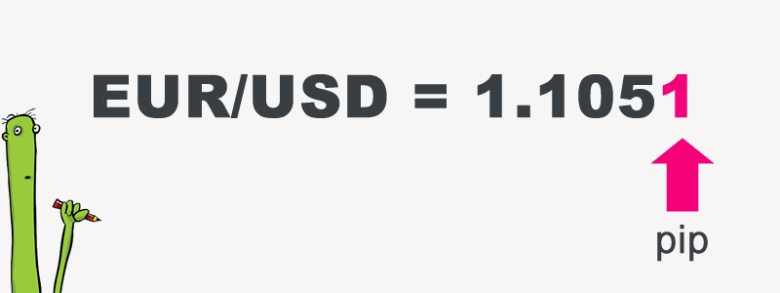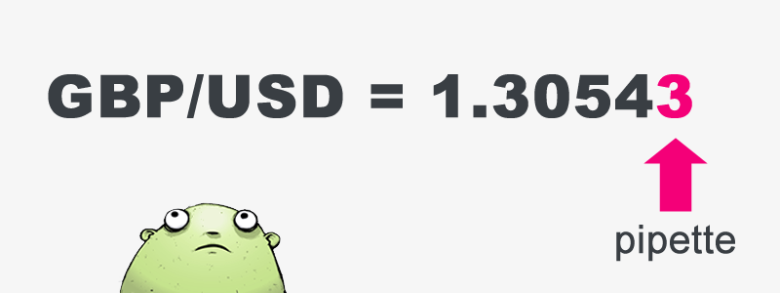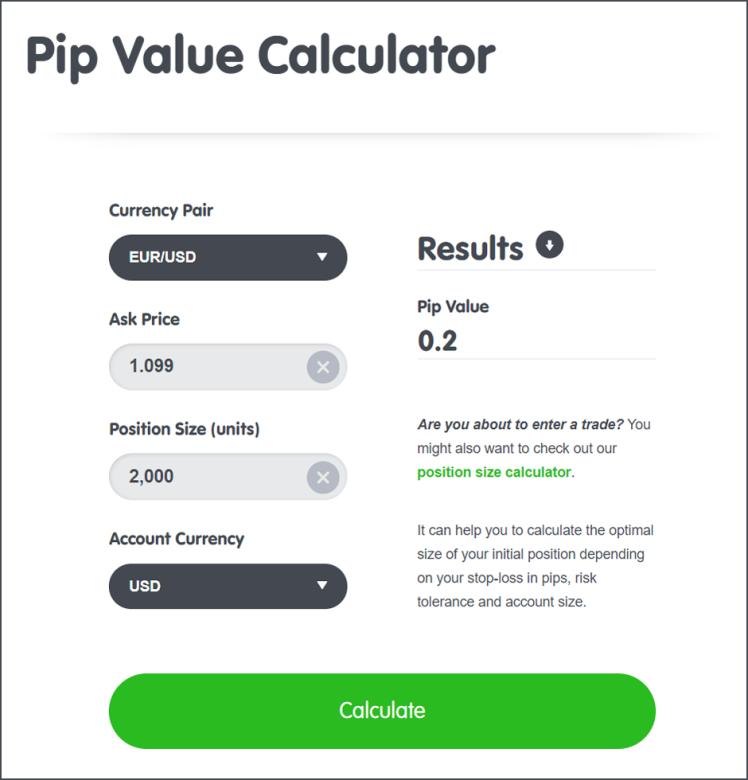Partner Center Find a Broker

A pip is the smallest value change in a currency pair’s exchange rate.

In forex trading, since currency prices typically move in tiny increments, they are quoted in a standardized unit called?pips.

One hundred pips is a “big figure”.

Pips are (usually) the smallest amount by which a currency quote can change.

When trading currencies, pips are the most basic unit of measure.

Just like a unit of measure for liquids is “ounces”, the unit of measure for currency quotes is “pips”.

Traders often use pips to reference profits or losses.

This means that the trader profited by 90 pips. The actual cash amount this represents depends on the pip value.

Even though a pip is a very small unit of measurement, forex traders are usually highly leveraged which means even a single pip move in price can mean huge profit or loss.

For instance, if the EUR/USD moves from 1.2250 to 1.2251, that is ONE pip.A pip is usually the last decimal place of a price quote.

Most pairs go out to 4 decimal places, but there are some exceptions like Japanese yen pairs (they go out to two decimal places).

For example, for EUR/USD, it is?0.0001, and for USD/JPY, it is?0.01.

There are forex brokers that quote currency pairs beyond the standard “4 and 2” decimal places to “5 and 3” decimal places.

They are quoting?FRACTIONAL PIPS, also called “pipettes.”

There are 10 fractional pips to a whole pip.

For instance, if GBP/USD moves from 1.30542?to 1.30543,?that .00001 USD move higher is?ONE PIPETTE.What does “pip” stand for?

Some forex trading platforms say pip stands for “percentage in point“, while others say it stands for “price interest point“.

When discussing price movements, pips are used in the forex market. Points and ticks are used in the futures market.

## How to Calculate the Pip Value

The value of each pip depends on three factors:

1. The currency pair being traded.
2. The size of the trade
3. The exchange rate.

Based on these factors, the fluctuation of even a single pip can have a huge impact on the value of your trade position.

### Pip Value Calculation Example

A \$50,000 trade involving the USD/CAD pair is closed at 1.3050 after gaining 50 pips.

To calculate the profit in U.S. dollars, complete the following three steps:

#### 1. Determine the number of quote currency (CAD) each pip represents.

Multiply the amount of the trade by 1 pip:

`50,000 x 0.0001 = 5 CAD per pip`

#### 2. Calculate the number of base currency (USD) per pip.

Divide the number of CAD per pip (from step 1) by the exchange rate to arrive at the number of USD per pip:

`5 ÷ 1.3050 = 3.83 USD per pip`

#### 3. Determine the total profit or loss of the trade.

Multiply the number of pips gained, by the value of each pip in USD (from step 2) to arrive at the total profit/loss for the trade:

`50 (pips gained) x 3.83 = \$191.50 USD profit`

## Pip Value Calculator

If you’re lazy, we have just the tool for you!